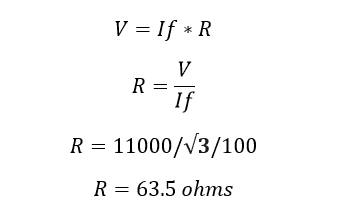# How to calculate NGR resistance value?

## How to calculate the NGR value?

NGR- Neutral grounding resistor. NGR is used to limit the fault current in a generator or a transformer during earth faults. The following factor should be considered before selecting the NGR resistance value.

1. The fault current should be kept in mind while deciding on the NGR value. Too high a value of the NGR would limit the current to a very low value which will not be able to activate the earth fault protection.
2. The system capacitance should be taken care, the NGR should discharge the capacitor during the earth fault. Otherwise, the charged capacitor creates transient overvoltages.
3. System voltage should be considered

### How to calculate NGR resistance value?

Consider the maximum fault current flow through the resistor is 100 Amps. Therefore the current carrying capacity of the resistance is 100 Amps. The system voltage V is 11kV. The phase to ground voltage is 11kV/ √3 Apply ohms law..The NGR resistance value should be 63.5 Ohms for 100 Amps Fault current

1.Electrical concepts

Nice article

2.Bikash das

Can u calculate the NGR value of 30 mva alternator

•electrical

yes you can calculate, for that we need nominal voltage and current

3.محمد

THANKS